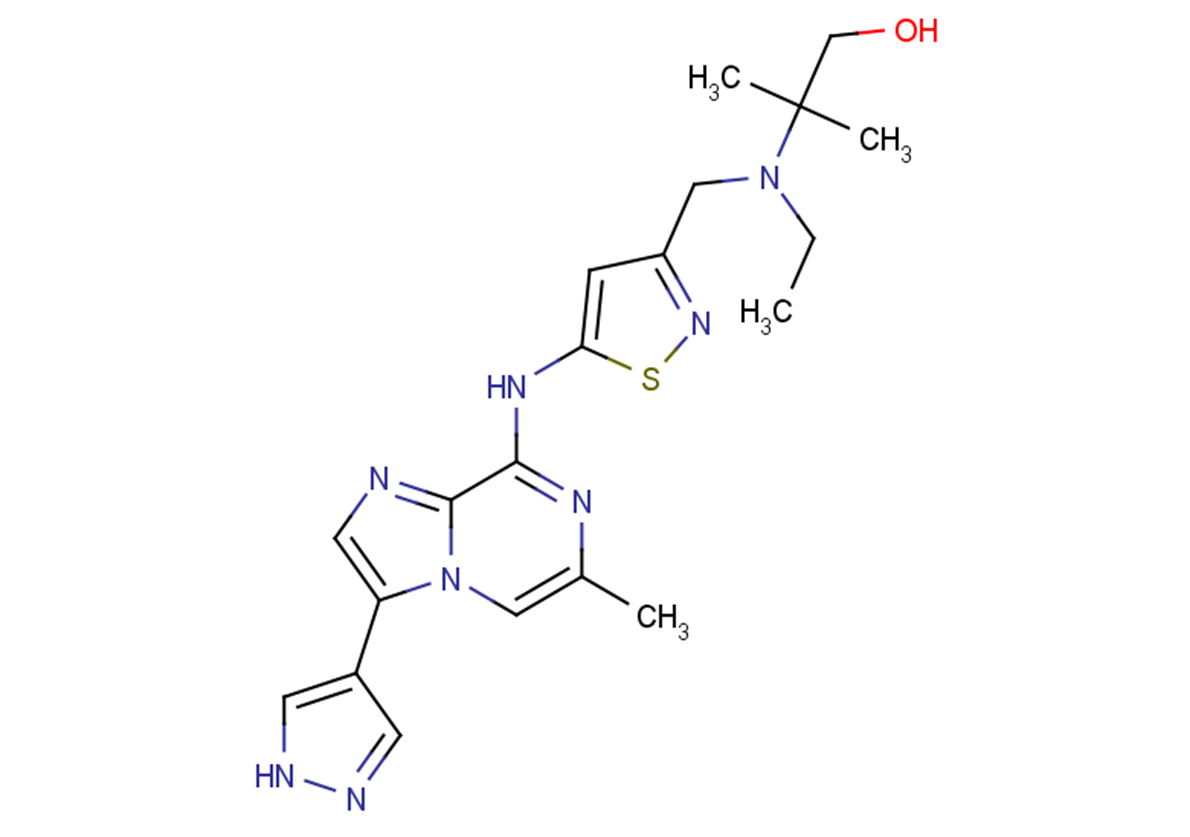# SCH-1473759

Catalog No. T16862   CAS 1094069-99-4

SCH-1473759 is an inhibitor of the aurora (IC50s: 4 and 13 nM for Aurora A and B, respectively).

All products from TargetMol are for Research Use Only. Not for Human or Veterinary or Therapeutic Use.SCH-1473759, CAS 1094069-99-4
Product consultation
Get quote
Purity: 98%
Biological Description
Chemical Properties
Storage & Solubility Information
 Description SCH-1473759 is an inhibitor of the aurora (IC50s: 4 and 13 nM for Aurora A and B, respectively). Targets&IC50 Aurora A:4 nM, Aurora B:13 nM In vitro SCH-1473759 inhibits HCT116 cells proliferation (IC50: 6 nM). SCH 1473759 inhibits tumor cell lines from different tissues (breast, ovarian, prostate, lung, colon, brain, gastric, renal, skin, and leukemia). SCH-1473759 also inhibits the Src family of kinases (IC50<10 nM), Chk1 (IC50=13 nM), VEGFR2 (IC50=1 nM), and IRAK4 (IC50=37 nM). SCH-1473759 directly binds to aurora A and B with Kds of 20 and 30 nM, respectively. It does not have significant activity (IC50>1000 nM) against 34 other kinases representing different families of the kinome. The most sensitive cell lines include A2780, LNCap, N87, Molt4, K562, and CCRF-CEM with IC50 values <5 nM . In vivo SCH-1473759 shows good exposure in all species with the clearance being high in rodents and moderate in dog and monkey. SCH 1473759 dose- and schedule-dependent anti-tumor activity in four human tumor xenograft models. SCH-1473759 at a low dose of 5 mg/kg (i.p., bid) is well-tolerated in a continuous dosing schedule and shows 50% tumor growth inhibition(TGI) on day 16. A higher dose of 10mg/kg(i.p., bid) is well-tolerated in an intermittent schedule (5 days on, 5 days off) and gave 69% TGI on day 16. The half-life is also moderate, but tissue distribution is high .
 Molecular Weight 426.54 Formula C20H26N8OS CAS No. 1094069-99-4

#### Storage

Powder: -20°C for 3 years

In solvent: -80°C for 2 years

#### Solubility Information

( < 1 mg/ml refers to the product slightly soluble or insoluble )

##Dose Conversion

You can also refer to dose conversion for different animals. More

##In vivo Formulation Calculator (Clear solution)

Step One: Enter information below
Dosage
mg/kg
Average weight of animals
g
Dosing volume per animal
ul
Number of animals
Step Two: Enter the in vivo formulation
% DMSO
%
% Tween 80
% ddH2O

##Calculator

Molarity Calculator
Dilution Calculator
Reconstitution Calculation
Molecular Weight Calculator
=
X
X

### Molarity Calculator allows you to calculate the

• Mass of a compound required to prepare a solution of known volume and concentration
• Volume of solution required to dissolve a compound of known mass to a desired concentration
• Concentration of a solution resulting from a known mass of compound in a specific volume
See Example

An example of a molarity calculation using the molarity calculator
What is the mass of compound required to make a 10 mM stock solution in 10 ml of water given that the molecular weight of the compound is 197.13 g/mol?
Enter 197.13 into the Molecular Weight (MW) box
Enter 10 into the Concentration box and select the correct unit (millimolar)
Enter 10 into the Volume box and select the correct unit (milliliter)
Press calculate
The answer of 19.713 mg appears in the Mass box

X
=
X

### Calculator the dilution required to prepare a stock solution

Calculate the dilution required to prepare a stock solution
The dilution calculator is a useful tool which allows you to calculate how to dilute a stock solution of known concentration. Enter C1, C2 & V2 to calculate V1.

See Example

An example of a dilution calculation using the Tocris dilution calculator
What volume of a given 10 mM stock solution is required to make 20ml of a 50 μM solution?
Using the equation C1V1 = C2V2, where C1=10 mM, C2=50 μM, V2=20 ml and V1 is the unknown:
Enter 10 into the Concentration (start) box and select the correct unit (millimolar)
Enter 50 into the Concentration (final) box and select the correct unit (micromolar)
Enter 20 into the Volume (final) box and select the correct unit (milliliter)
Press calculate
The answer of 100 microliter (0.1 ml) appears in the Volume (start) box

=
/

### Calculate the volume of solvent required to reconstitute your vial.

The reconstitution calculator allows you to quickly calculate the volume of a reagent to reconstitute your vial.
Simply enter the mass of reagent and the target concentration and the calculator will determine the rest.

g/mol

### Enter the chemical formula of a compound to calculate its molar mass and elemental composition

Tip: Chemical formula is case sensitive: C10H16N2O2 c10h16n2o2

Instructions to calculate molar mass (molecular weight) of a chemical compound:
To calculate molar mass of a chemical compound, please enter its chemical formula and click 'Calculate'.
Definitions of molecular mass, molecular weight, molar mass and molar weight:
Molecular mass (molecular weight) is the mass of one molecule of a substance and is expressed n the unified atomic mass units (u). (1 u is equal to 1/12 the mass of one atom of carbon-12)
Molar mass (molar weight) is the mass of one mole of a substance and is expressed in g/mol.

bottom

## Tech Support

Please see Inhibitor Handling Instructions for more frequently ask questions. Topics include: how to prepare stock solutions, how to store products, and cautions on cell-based assays & animal experiments, etc.